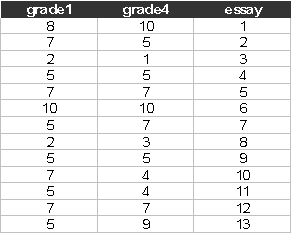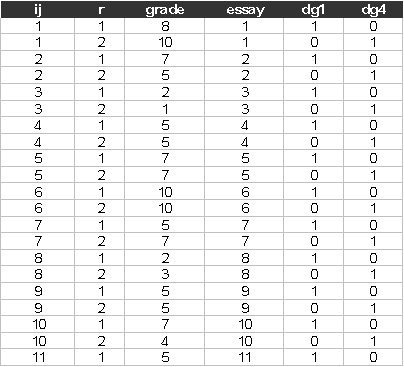Exercise C1, linear model

Original data description (grader1.dat)

Number of observations (rows): 198

Number of variables (columns): 3

Variables:

grade1= grade awarded by grader 1 {1,2,…,10)

grade4=grade awarded by grader 4 {1,2,….,10}

essay=essay identifier

The first few lines of the grader1.dat look like:To use the data in Sabre we need to stack the data, with grade1 and grade4 as a single column grade. We have done this for you and generated an identifier to distinguish grade1 and grade4, i.e. dg4=1, if grade4 =1 and 0 otherwise.

Data description (grader2.dat)

Number of observations (rows): 396

Number of variables (columns): 6

Variables:

ij = essay identifier (1,2,…,198)

r = response (1,2)

grade= grade awarded

essay= essay identifier  (this is a copy of ij)

dg1 = 1 if this is the grade from grader 1, 0 otherwise

dg4 = 1 if this is the grade from grader 4, 0 otherwise

The first few lines of the stacked data (grader2.dat) look like:Start Sabre and specify transcript file:

out grader2.log

data ij r grade essay dg1 dg4

read grader2.dat

Suggested exercise:

(1) Estimate the linear model using Sabre on grade, with just a constant and no other effects;

(2) Estimate the linear model, allowing for the essay random effect, use mass 64, this is a linear model and a lot of quadrature points are need to get a good approximation to the integrated likelihood. Are the essay effects significant? What impact do they have on the model?

(3) Re-estimate the linear model allowing for both the essay random effect and dg4.

(4) How do the results change as compared to a model with just a constant? Interpret your results.

References

Johnson, V. E., and Albert, J., H., (1999), Ordinal Data Modelling, Springer, New York.

Rabe-Hesketh, S., and Skrondal, A., (2005), Multilevel and Longitudinal Modelling using Stata, Stata Press, Stata Corp, College Station, Texas.